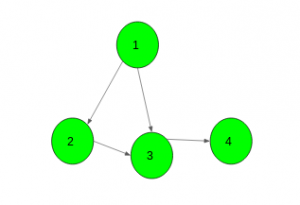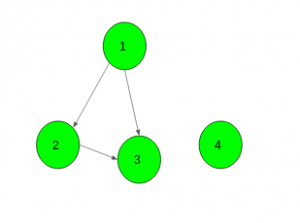# Check if a directed graph is connected or not

Given a directed graph. The task is to check if the given graph is connected or not.

Examples:

Input:Output: Yes

Input:Output: No

## Recommended: Please try your approach on {IDE} first, before moving on to the solution.

Approach:

1. Take two bool arrays vis1 and vis2 of size N (number of nodes of a graph) and keep false in all indexes.
2. Start at a random vertex v of the graph G, and run a DFS(G, v).
3. Make all visited vertices v as vis1[v] = true.
4. Now reverse the direction of all the edges.
5. Start DFS at the vertex which was chosen at step 2.
6. Make all visited vertices v as vis2[v] = true.
7. If any vertex v has vis1[v] = false and vis2[v] = false then the graph is not connected.

Below is the implementation of the above approach:

## C++

 `// C++ implementation of the approach ` `#include ` `using` `namespace` `std; ` `#define N 100000 ` ` `  `// To keep correct and reverse direction ` `vector<``int``> gr1[N], gr2[N]; ` ` `  `bool` `vis1[N], vis2[N]; ` ` `  `// Function to add edges ` `void` `Add_edge(``int` `u, ``int` `v) ` `{ ` `    ``gr1[u].push_back(v); ` `    ``gr2[v].push_back(u); ` `} ` ` `  `// DFS function ` `void` `dfs1(``int` `x) ` `{ ` `    ``vis1[x] = ``true``; ` ` `  `    ``for` `(``auto` `i : gr1[x]) ` `        ``if` `(!vis1[i]) ` `            ``dfs1(i); ` `} ` ` `  `// DFS function ` `void` `dfs2(``int` `x) ` `{ ` `    ``vis2[x] = ``true``; ` ` `  `    ``for` `(``auto` `i : gr2[x]) ` `        ``if` `(!vis2[i]) ` `            ``dfs2(i); ` `} ` ` `  `bool` `Is_Connected(``int` `n) ` `{ ` `    ``// Call for correct direction ` `    ``memset``(vis1, ``false``, ``sizeof` `vis1); ` `    ``dfs1(1); ` ` `  `    ``// Call for reverse direction ` `    ``memset``(vis2, ``false``, ``sizeof` `vis2); ` `    ``dfs2(1); ` ` `  `    ``for` `(``int` `i = 1; i <= n; i++) { ` ` `  `        ``// If any vertex it not visited in any direction ` `        ``// Then graph is not connected ` `        ``if` `(!vis1[i] and !vis2[i]) ` `            ``return` `false``; ` `    ``} ` ` `  `    ``// If graph is connected ` `    ``return` `true``; ` `} ` ` `  `// Driver code ` `int` `main() ` `{ ` `    ``int` `n = 4; ` ` `  `    ``// Add edges ` `    ``Add_edge(1, 2); ` `    ``Add_edge(1, 3); ` `    ``Add_edge(2, 3); ` `    ``Add_edge(3, 4); ` ` `  `    ``// Function call ` `    ``if` `(Is_Connected(n)) ` `        ``cout << ``"Yes"``; ` `    ``else` `        ``cout << ``"No"``; ` ` `  `    ``return` `0; ` `} `

## Java

 `// Java implementation of the approach ` `import` `java.util.*; ` ` `  `class` `GFG  ` `{ ` `    ``static` `int` `N = ``100000``; ` ` `  `    ``// To keep correct and reverse direction ` `    ``@SuppressWarnings``(``"unchecked"``) ` `    ``static` `Vector[] gr1 = ``new` `Vector[N]; ` `    ``@SuppressWarnings``(``"unchecked"``) ` `    ``static` `Vector[] gr2 = ``new` `Vector[N]; ` ` `  `    ``static` `boolean``[] vis1 = ``new` `boolean``[N]; ` `    ``static` `boolean``[] vis2 = ``new` `boolean``[N]; ` ` `  `    ``static` `{ ` `        ``for` `(``int` `i = ``0``; i < N; i++) ` `        ``{ ` `            ``gr1[i] = ``new` `Vector<>(); ` `            ``gr2[i] = ``new` `Vector<>(); ` `        ``} ` `    ``} ` ` `  `    ``// Function to add edges ` `    ``static` `void` `Add_edge(``int` `u, ``int` `v) ` `    ``{ ` `        ``gr1[u].add(v); ` `        ``gr2[v].add(u); ` `    ``} ` ` `  `    ``// DFS function ` `    ``static` `void` `dfs1(``int` `x) ` `    ``{ ` `        ``vis1[x] = ``true``; ` `        ``for` `(``int` `i : gr1[x]) ` `            ``if` `(!vis1[i]) ` `                ``dfs1(i); ` `    ``} ` ` `  `    ``// DFS function ` `    ``static` `void` `dfs2(``int` `x)  ` `    ``{ ` `        ``vis2[x] = ``true``; ` `        ``for` `(``int` `i : gr2[x]) ` `            ``if` `(!vis2[i]) ` `                ``dfs2(i); ` `    ``} ` ` `  `    ``static` `boolean` `Is_connected(``int` `n) ` `    ``{ ` ` `  `        ``// Call for correct direction ` `        ``Arrays.fill(vis1, ``false``); ` `        ``dfs1(``1``); ` ` `  `        ``// Call for reverse direction ` `        ``Arrays.fill(vis2, ``false``); ` `        ``dfs2(``1``); ` ` `  `        ``for` `(``int` `i = ``1``; i <= n; i++) ` `        ``{ ` ` `  `            ``// If any vertex it not visited in any direction ` `            ``// Then graph is not connected ` `            ``if` `(!vis1[i] && !vis2[i]) ` `                ``return` `false``; ` `        ``} ` ` `  `        ``// If graph is connected ` `        ``return` `true``; ` `    ``} ` ` `  `    ``// Driver Code ` `    ``public` `static` `void` `main(String[] args) ` `    ``{ ` `        ``int` `n = ``4``; ` ` `  `        ``// Add edges ` `        ``Add_edge(``1``, ``2``); ` `        ``Add_edge(``1``, ``3``); ` `        ``Add_edge(``2``, ``3``); ` `        ``Add_edge(``3``, ``4``); ` ` `  `        ``// Function call ` `        ``if` `(Is_connected(n)) ` `            ``System.out.println(``"Yes"``); ` `        ``else` `            ``System.out.println(``"No"``); ` `    ``} ` `} ` ` `  `// This code is contributed by ` `// sanjeev2552 `

## Python3

 `# Python3 implementation of the approach  ` `N ``=` `100000` ` `  `# To keep correct and reverse direction  ` `gr1 ``=` `{}; gr2 ``=` `{};  ` ` `  `vis1 ``=` `[``0``] ``*` `N; vis2 ``=` `[``0``] ``*` `N;  ` ` `  `# Function to add edges  ` `def` `Add_edge(u, v) :  ` ` `  `    ``if` `u ``not` `in` `gr1 : ` `        ``gr1[u] ``=` `[]; ` `         `  `    ``if` `v ``not` `in` `gr2 : ` `        ``gr2[v] ``=` `[]; ` `         `  `    ``gr1[u].append(v); ` `    ``gr2[v].append(u);  ` ` `  `# DFS function  ` `def` `dfs1(x) :  ` `    ``vis1[x] ``=` `True``; ` `    ``if` `x ``not` `in` `gr1 : ` `        ``gr1[x] ``=` `{}; ` `         `  `    ``for` `i ``in` `gr1[x] : ` `        ``if` `(``not` `vis1[i]) : ` `            ``dfs1(i)  ` ` `  `# DFS function  ` `def` `dfs2(x) :  ` ` `  `    ``vis2[x] ``=` `True``;  ` ` `  `    ``if` `x ``not` `in` `gr2 : ` `        ``gr2[x] ``=` `{}; ` `         `  `    ``for` `i ``in` `gr2[x] :  ` `        ``if` `(``not` `vis2[i]) : ` `            ``dfs2(i);  ` ` `  `def` `Is_Connected(n) :  ` ` `  `    ``global` `vis1; ` `    ``global` `vis2; ` `     `  `    ``# Call for correct direction ` `    ``vis1 ``=` `[``False``] ``*` `len``(vis1); ` `    ``dfs1(``1``); ` `     `  `    ``# Call for reverse direction ` `    ``vis2 ``=` `[``False``] ``*` `len``(vis2); ` `    ``dfs2(``1``); ` `     `  `    ``for` `i ``in` `range``(``1``, n ``+` `1``) : ` `         `  `        ``# If any vertex it not visited in any direction ` `        ``# Then graph is not connected ` `        ``if` `(``not` `vis1[i] ``and` `not` `vis2[i]) : ` `            ``return` `False``; ` `             `  `    ``# If graph is connected ` `    ``return` `True``;  ` ` `  `# Driver code  ` `if` `__name__ ``=``=` `"__main__"` `:  ` ` `  `    ``n ``=` `4``;  ` ` `  `    ``# Add edges  ` `    ``Add_edge(``1``, ``2``);  ` `    ``Add_edge(``1``, ``3``);  ` `    ``Add_edge(``2``, ``3``);  ` `    ``Add_edge(``3``, ``4``);  ` ` `  `    ``# Function call  ` `    ``if` `(Is_Connected(n)) : ` `        ``print``(``"Yes"``);  ` `    ``else` `: ` `        ``print``(``"No"``);  ` ` `  `# This code is contributed by AnkitRai01 `

## C#

 `// C# implementation of the approach ` `using` `System; ` `using` `System.Collections.Generic; ` ` `  `class` `GFG  ` `{ ` `    ``static` `int` `N = 100000; ` ` `  `    ``// To keep correct and reverse direction ` `    ``static` `List<``int``>[] gr1 = ``new` `List<``int``>[N]; ` ` `  `    ``static` `List<``int``>[] gr2 = ``new` `List<``int``>[N]; ` ` `  `    ``static` `bool``[] vis1 = ``new` `bool``[N]; ` `    ``static` `bool``[] vis2 = ``new` `bool``[N]; ` ` `  `    ``// Function to add edges ` `    ``static` `void` `Add_edge(``int` `u, ``int` `v) ` `    ``{ ` `        ``gr1[u].Add(v); ` `        ``gr2[v].Add(u); ` `    ``} ` ` `  `    ``// DFS function ` `    ``static` `void` `dfs1(``int` `x) ` `    ``{ ` `        ``vis1[x] = ``true``; ` `        ``foreach` `(``int` `i ``in` `gr1[x]) ` `            ``if` `(!vis1[i]) ` `                ``dfs1(i); ` `    ``} ` ` `  `    ``// DFS function ` `    ``static` `void` `dfs2(``int` `x)  ` `    ``{ ` `        ``vis2[x] = ``true``; ` `        ``foreach` `(``int` `i ``in` `gr2[x]) ` `            ``if` `(!vis2[i]) ` `                ``dfs2(i); ` `    ``} ` ` `  `    ``static` `bool` `Is_connected(``int` `n) ` `    ``{ ` ` `  `        ``// Call for correct direction ` `        ``for` `(``int` `i = 0; i < n; i++) ` `            ``vis1[i] = ``false``; ` `        ``dfs1(1); ` ` `  `        ``// Call for reverse direction ` `        ``for` `(``int` `i = 0; i < n; i++) ` `            ``vis2[i] = ``false``; ` `        ``dfs2(1); ` ` `  `        ``for` `(``int` `i = 1; i <= n; i++) ` `        ``{ ` ` `  `            ``// If any vertex it not visited in any direction ` `            ``// Then graph is not connected ` `            ``if` `(!vis1[i] && !vis2[i]) ` `                ``return` `false``; ` `        ``} ` ` `  `        ``// If graph is connected ` `        ``return` `true``; ` `    ``} ` ` `  `    ``// Driver Code ` `    ``public` `static` `void` `Main(String[] args) ` `    ``{ ` `        ``int` `n = 4; ` `        ``for` `(``int` `i = 0; i < N; i++) ` `        ``{ ` `            ``gr1[i] = ``new` `List<``int``>(); ` `            ``gr2[i] = ``new` `List<``int``>(); ` `        ``} ` `         `  `        ``// Add edges ` `        ``Add_edge(1, 2); ` `        ``Add_edge(1, 3); ` `        ``Add_edge(2, 3); ` `        ``Add_edge(3, 4); ` ` `  `        ``// Function call ` `        ``if` `(Is_connected(n)) ` `            ``Console.WriteLine(``"Yes"``); ` `        ``else` `            ``Console.WriteLine(``"No"``); ` `    ``} ` `} ` ` `  `// This code is contributed by PrinciRaj1992 `

Output:

```Yes
```

Attention reader! Don’t stop learning now. Get hold of all the important DSA concepts with the DSA Self Paced Course at a student-friendly price and become industry ready.

My Personal Notes arrow_drop_uppawanasipugmailcom

If you like GeeksforGeeks and would like to contribute, you can also write an article using contribute.geeksforgeeks.org or mail your article to contribute@geeksforgeeks.org. See your article appearing on the GeeksforGeeks main page and help other Geeks.

Please Improve this article if you find anything incorrect by clicking on the "Improve Article" button below.

Article Tags :
Practice Tags :

2

Please write to us at contribute@geeksforgeeks.org to report any issue with the above content.